SELF 38 S.B. Karavashkin and O.N. KaravashkinaAnd not only ambiguity and imaginary non-simultaneity tell us that the Lorentz transformation is incorrect. To make sure, let us on the basis of (15) and (16) see how the time intervals will transform in passing from the stationary frame to that moving. Let us choose two values of time in the stationary frame, t1 and  t2 , and determine for each end of both rods the time intervals dependently of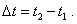(19)
 For the rod AB we yield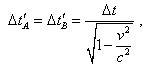(20)
 and for the rod A'B'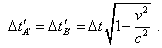(21)
 As we can see, the time intervals are equal for the points that move synchronously or are at rest with respect to frame. But the time transformation is different dependently of the pattern of motion of the material point. This is unnaturally, of course, for inertial frames, and evidences of incorrect Lorentz transformation, as the pace of time in one frame is one for the whole frame, in limits of relativistic conception also. But if, dependently on the state of motion, the pace of time for each material point is different, this evidences of incorrect transformation. To see the general pattern of regularity of time transformation between the frames in the relativistic conception, consider additionally some material point D that moves with the speed u in the positive direction of axis x of the stationary frame. The plot of its motion in the 4-D Minkowski space in the stationary frame is described by the equation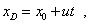(22)
 where x0 is the location of material point at the initial moment of time t = 0 . Applying to (22) the conventional Lorentz transformation (14), we yield the expressions describing the trajectory in the moving frame. The equations will be such: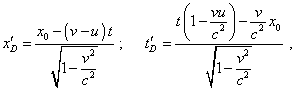(23)
 hence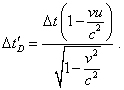(24)
 We see from (24) that in general case, too, the transformation of time interval, in accordance with Lorentz transformation, depends on the pattern of motion of material point, and the transformation coefficient can be more and less than 1, dependently on direction of motion of the material point. We would like to mark again, the time interval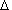t'D is not a natural time interval for this material point. According to the relativistic understanding, this time interval determines the speed of change of the point’s location with respect to the moving frame, and if in this frame there will be an infinite number of material points moving with different speeds in different directions, then, according to the Lorentz transformation, we will have an infinite number of transformations of time interval of the very moving frame, which makes this interval indefinite. And this will serve an insurmountable obstacle to establish the physical time in this frame, which contradicts the statement of problem.

Contents: / 28 / 29 / 30 / 31 / 32 / 33 / 34 / 35 / 36 / 37 / 38 / 39 / 40 / 41 / 42 /